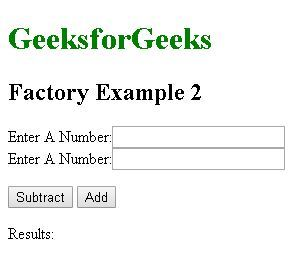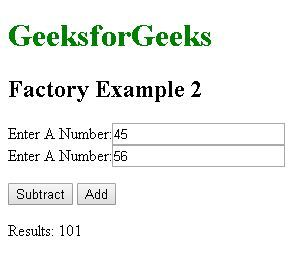# AngularJS | Factory Method

AngularJS Factory Method makes the development process of AngularJS application more robust. A factory is a simple function which allows us to add some logic to a created object and return the created object. The factory is also used to create/return a function in the form of reusable code which can be used anywhere within the application. Whenever we create an object using factory it always returns a new instance for that object. The object returned by the factory can be integrated(injectible) with different components of the Angularjs framework such as controller, service, filter or directive.

Use:
In practical Scenario Factory generally acts as container or class for a collection of functions which fulfills different features of the application. When using with a constructor function it can be initiated within different Controllers.

Syntax:

`module.factory( 'factoryName', function(){ Custom code....});`

Example:
The Following example illustrate the use of factory code instantiated inside a controller to generate a random number

 ` ` `<``html``> ` ` `  `<``head``> ` `    ``<``title``>Factory Example 1 ` ` `  `    ``<``script` `src``= ` `"https://ajax.googleapis.com/ajax/libs/angularjs/1.6.9/angular.min.js"``> ` `    `` ` `    ``<``script``> ` `        ``var application = angular.module('myApp', []); ` ` `  `        ``application.factory('random', function() { ` `            ``var randomObject = {}; ` `            ``var number = Math.floor(Math.random() * 100); ` `            ``randomObject.generate = function() { ` `                ``return number; ` `            ``}; ` `            ``return randomObject; ` `        ``}); ` `       `  `        ``application.controller('thisapp', function(\$scope, random) { ` `            ``\$scope.generateRandom = function() { ` `                ``\$scope.randomNumber = random.generate(); ` `            ``}; ` `        ``}); ` `    `` ` ` ` ` `  `<``body``> ` `    ``<``h1` `style``=``"color:green"``>GeeksforGeeks ` `    ``<``h2``>Factory Examples ` `    ``<``div` `ng-app``=``"myApp"` `ng-controller``=``"thisapp"``> ` `        ``<``button` `ng-click``=``"generateRandom()"``> ` `          ``Generate Random Number ` `      `` ` `        ``<``br``>{{randomNumber}} ` `    `` ` ` ` ` `  ` `

Output:On Clicking the generate random number button we get a different number every time. In this example, we use the factory method to define a function which carries a variable and using the Math.random we store a random value to that variable every time this function is called. This function is then called in the controller whose \$scope variable carries the random value from the called function we then call this controller to our HTML code to display the result.

Example: This example make use of factory to create a function to find addition or subtraction of two numbers. this function is then loaded in the controller \$scope variable which passes them to the html code for displaying the results.

 ` ` `<``html``> ` ` `  `<``head``> ` `    ``<``title``>Factory Example 2 ` `    ``<``script` `src``= ` `"https://ajax.googleapis.com/ajax/libs/angularjs/1.6.9/angular.min.js"``> ` `    `` ` `    ``<``script``> ` `        ``var application = angular.module('myApp', []); ` ` `  `        ``application.factory('MyFactoryService', function() { ` `            ``var factory = {}; ` `            ``factory.Subtract = function(a, b) { ` `                ``return a - b; ` `            ``}; ` `            ``factory.Add = function(a, b) { ` `                ``return a + b; ` `            ``}; ` `            ``return factory; ` `        ``}); ` ` `  `        ``application.controller('thisapp', function( ` `                               ``\$scope, MyFactoryService) { ` `            ``\$scope.result = function() { ` `                ``\$scope.results =  ` `                  ``MyFactoryService.Subtract(\$scope.num1, \$scope.num2) ` `            ``}; ` `            ``\$scope.result2 = function() { ` `                ``\$scope.results =  ` `                  ``MyFactoryService.Add(\$scope.num1, \$scope.num2) ` `            ``}; ` `        ``}); ` `    `` ` ` ` ` `  `<``body``> ` `    ``<``h1` `style``=``"color:green"``>GeeksforGeeks ` `    ``<``h2``>Factory Example 2 ` `    ``<``div` `ng-app``=``"myApp"` `ng-controller``=``"thisapp"``> ` `        ``<``p``> ` `            ``Enter A Number: ` `            ``<``input` `type``=``"number"` `ng-model``=``"num1"` `/> ` `            ``<``br``/> Enter A Number: ` `            ``<``input` `type``=``"number"` `ng-model``=``"num2"` `/> ` `            ``<``br``/> ` `        `` ` `        ``<``button` `ng-click``=``"result()"``>Subtract ` `        ``<``button` `ng-click``=``"result2()"``>Add ` `        ``<``p``>Results: {{results}} ` `    `` ` ` ` ` `  ` `

Output:
Before:After entering the input clicking on the add/subtract button displays the result:My Personal Notes arrow_drop_upCheck out this Author's contributed articles.

If you like GeeksforGeeks and would like to contribute, you can also write an article using contribute.geeksforgeeks.org or mail your article to contribute@geeksforgeeks.org. See your article appearing on the GeeksforGeeks main page and help other Geeks.

Please Improve this article if you find anything incorrect by clicking on the "Improve Article" button below.

Article Tags :

Be the First to upvote.

Please write to us at contribute@geeksforgeeks.org to report any issue with the above content.